The Development of financial and economic efficiency of the enterprise on the basis of economic-mathematical modeling

UDC 338:517
Publication date: 27.02.2017
International Journal of Professional Science № 3-2017

The Development of financial and economic efficiency of the enterprise on the basis of economic-mathematical modeling

Balashova Raisa Ivanovna
doctor of Economics, associate Professor
Donetsk national technical University, Donetsk
Abstract: The article considers the possibility of applying economic-mathematical models constructed on the basis of economic analysis of the evidence and a methodological orientation to predict expected performance. It is shown that for the planning of labor productivity, the best relationship is linear model, followed by the logarithmic function, hyperbola and hyperbola with offset. It is concluded that the use of economic-mathematical models is relevant and provides the optimal actions necessary for economic development
Keywords: development, enterprise, efficiency, mathematical modeling, Finance, Economics

1. In the activity of enterprises in modern conditions of economic development the method of economic-mathematical modeling is used to evaluate and predict economic and financial results.

As a result of development of information technologies there is the opportunity to conduct a largely systematic process of modeling, which includes the creation of a model, interpretation of simulation results the statistical evaluation. The application software facilitates the construction of models that account for a significant number of internal and external factors of the enterprise.

Economic-mathematical model of activity and development of an enterprise is a reflection of her as a set of logical relations, equations, and graphs. The advantage of using such models lies in the possibility of obtaining with their help, we confirm the calculations of the insights about the General features of the future sustainable development of the enterprise.

Development of economic-mathematical model for the enterprise will allow to define the sequence of possible state – trajectories of development that cannot be considered as strictly determinirovannyi, tightly regulating its activity. They describe only the scenarios of its development, asking fundamentally possible way, the overall Outlook of activities of enterprises subordinated to the achievement of strategic objectives. Therefore, when forming long-term plans for the company needs to develop several options.

For economic and mathematical modeling of enterprise can be applied the method of dynamic simulation, including techniques of formalization of the management of financial-economic activity of the enterprise. This method allows to structure the formation of enterprise development, to describe and predict the process of sustainable development, covering a large number of variables.

2.Materials and methods. Theoretical and methodological basis of the study of economic theory, the writings of scholars. The information base consists of legislative acts concerning the activities of enterprises, statistical materials of the State statistics service. The study used General scientific methods, methods of abstraction and complexity, methods of comparison and generalization, the method of mathematical modeling.

The results of formalizing the development of the enterprise is envisaged to be meaningful and comprehensive, but at the same time as simple as possible. A large number of characteristics in the formalization of enterprise development selects only those that are based on theoretical assumptions considered to be the primary and correspond to the task of modeling. A key role in the description of sustainable development of the company during its formalization is the choice of initial reserves, the nature, the methods applied and the scope of services in a predictable period of time based on the results of assessment of company potential.

The use of economic-mathematical models constructed on the basis of economic analysis of the evidence and a methodological orientation to predict expected performance is completely justified. In the course of creation ekonomiko-mathematical model and simulation algorithm a number of features inherent in the phenomenon are not taken into account fully, a number of parameters are inaccurate, sometimes it is unknown or uncertain values using average empirical values, etc. This eliminates the possibility of constructing a mathematical model that would correspond to reality, and there is a need to adopt and explore not one, but multiple models that reflect different possible changes in the forecast data.

However, the selected characteristics must be measured. Various options for the development of the enterprise should be reflected in the calculation of a certain set of economic indicators that would allow quantitative and qualitative evaluation of alternatives (measures) of development. To determine the impact of enterprise development on the results of financial and economic activities necessary to build optimization models linear programming.

The use of mathematical modeling would be useful for future planning and forecasting indicators for the accumulation of resources of the enterprises so that the generated economic potential provided its sustainable development.

The relevance of the application of methods of economic-mathematical modeling on the basis of statistical analysis, confirm that to evaluate current performance and predict future currently used mainly methods of economic analysis. Thus, the development of modeling methods and statistical data processing in the practice of analysis corresponds to the modern requirements.

Problems of application of such modeling and analysis have been investigated by scientists and are reflected in the literature.

Says E. Pitman, one of the important tasks when creating a model is its identification, verification of its adequacy, the choice of its best forms. The identification problem consists in determining the parameters of the model on the basis of experimental data obtained in the result of observation of the studied phenomenon. For this purpose, data about the object of study, resulting in forming hypotheses about the model [1, p. 28].

As pointed out by M. Kubanin, the same trend can be expressed in several types of models, and each requires the definition of parameters. In practice this is usually done using least squares methods and maximum likelihood. Identification involved determining the fit of the model to experimental data, cannot always be made, and therefore empirical estimates [2, p. 12].

n the works of A. A. Gorchakov, A. A., Castle notes that the choice of the final model is the most important stage of the modelling process, where the problem of finding the optimal parameters between model complexity, completeness characteristics, and their accuracy [3, p. 73]. The most consistent can be considered as a systematic approach to a specified array, on the basis of which the principle of combination of the simplicity of the model and its adequacy. This principle requires a comparison of the results of the calculations to errors caused by various reasons and random nature of the results of the study, the incomplete correspondence of the model and of the object, the inaccuracy of the original data.

The works of A. I. Kharlamov, R. A. Shmoilova, A. P. Kulicheva indicated that the advantage of a particular model should be evaluated on the basis of statistical criteria taking into account the assessments of adequacy [4, p. 89; 5, p. 67].

The study of methods of economic-mathematical modeling and statistical analysis and the rationale for their use of activities of enterprises, the definition and method of determining the final form of the mathematical model of the efficiency factors is relevant to businesses of all types and forms of ownership.

The study of literary sources we can conclude that the sentences the selection procedure the final form of the economic-mathematical model of efficiency of activity of the enterprise is necessary to take into account the specifics of its activities. To solve the set task it is advisable to consider a number of statistical indicators. Checking adequacy of the model for calculating the economic enterprise development can be carried out on the basis of a number of statistical characteristics. Most of them are based on comparing the initial actual data and the theoretical values determined using the regression equation. Despite the fact that each of the characteristics it evaluates the same as a model of efficiency, the findings based on each of the equations do not always coincide, which implies the need to calculate the various estimates. Since, as a rule, uses several types of models, the analysis of those that are determined by the statistics (coefficients) becomes one of the most important tasks [6, p.12].

In the reviewed publications on economic modeling, the statistical characteristics are considered separately, attention is paid only to the parameters characterizing a specific model. If conclusions about the adequacy and reliability of models that characterize the performance of the enterprises, are the same, the problem is solved. But more often than not, and this part of the research model is particularly important for the economic practice of enterprises.

On the basis of the findings in the study of materials and economic activities of the nine campaigns (LLC «Stateb plus», LLC «Ilitash-opt», LLC «Darya-Avto», LLC «Fortuna», LLC «Guasar», LLC «Zet-prom» LLC «Crown», OOO «Lyuks-TRANS, OOO Dolomite plant», LLC «Ircomplex») used the method of economic-mathematical modeling:

1. Defined indicators ( ) that affect economic development t enterprise: productivity, the average cost of travel services, turnover, net income, cost of 1 RUB. of the gross income.

Table 1

These statistical coefficients according to the types of economic and mathematical models and economic indicators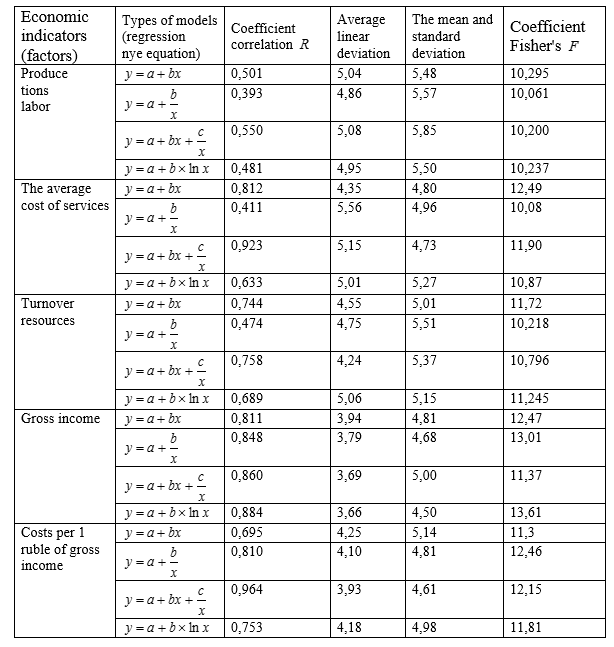2. The calculation data of the effectiveness of these measurements used a single-factor model four types:
– linear relationship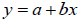;                             (1)
– inverse relationship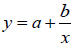;                          (2)
– hyperbole with an offset containing a linear and inverse component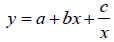;                                                                  (3)
– logarithmic function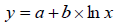;                     (4)
where  y – the efficiency coefficient, characterizing the activity of enterprises;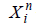– indicators of activity of the enterprises used in the calculation :
a, b, c – the parameters defined by the method of least squares for each of these models describing the dependence of the efficiency from changes in economic indicators of enterprises.
3. The analysis of the obtained statistical indicators using the correlation coefficient  R, the average linear deviation, standard deviation, coefficient Fisher’s  F.
From table 1 it follows that the correlation coefficient  , limit values which are within the range of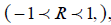reflects the relationship of each factor, economic indicator, economic growth. This figure indicates a significant correlation between economic development and the amount of income and the cost of 1 rub. of the gross income (value R approaching 1), slightly lower with turnover   R= 0,474-058 and quite weak, with labor productivity  R = 0,393-0,550.
Together with this General conclusion we may mention the differences in regression functions. If you analyze the volume of income deviation, maximum and minimum values of the correlation coefficient R for various functions irrelevant (they do not exceed 0,07), for the cost of 1 rub.  gross income the range of changes is from 0,7 to 0,95; for turnover of resources – from 0,47 to 0,76; the average cost of services from 0,41 to 0,92; for productivity – from 0,39 to 0,55. The use of these values allows to prefer one model or another, and also to organize models for the proximity of values to the real and to choose the optimal regression model.
A study of the average linear deviation defines the relative discrepancy between the actual and theoretical values of economic development activity. This ratio shows that the preference can be given to models with the lowest percentage of variance. For factor productivity average linear deviation changes from 4,86% (hyperbole) to 5,08% (hyperbole offset); average cost of services – from 4,35% (linear function) to 5,56% (hyperbole); the turnover of services – from 4,24 to 5,06% for gross income from the 3,66 to 3,94; for the cost of 1 rub  of income from 3,93% to 425%.
The data (table 2) indicate a considerable variation when considering the statistical parameter – standard deviation. It is known that, the smaller the value of this index, the more meaningful the equation. Thus, a linear function of assessment is best for productivity (5,48) and turnover resources (5,01), hyperbole shift (4,73) – average cost of services. The reliability of economic-mathematical model is determined by using the Fisher criterion: the reliability of the model increases with the increase of this indicator.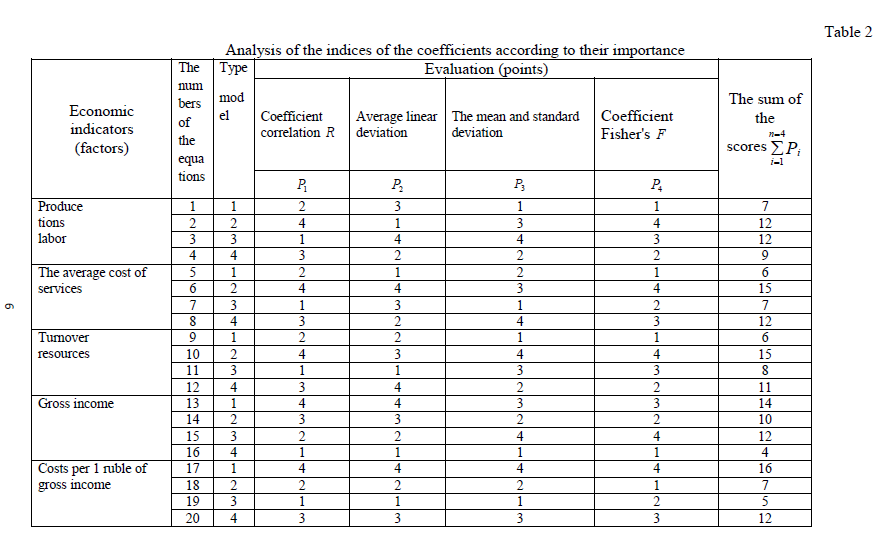All the values of the Fisher criterion exceeds the critical value, so the reliability of all these models is confirmed. Based on this characteristics you can analyze the data of economic-mathematical models and pick the best.
A comparison of the conclusions of models based on different performance indicators highlighted the divergence in the selected types of models.
4. The choice of an optimal model of efficiency of activity of enterprises on the basis of ranking obtained data of statistical indicators according to their importance. According to her determined for each model, the place it occupies on the basis of selected criteria. It will more specifically represent the importance of economic dependency for each indicator and to formulate the principle of determining the value of the model.
5. The formulation of the principle advantages of the model: characteristics of economic indicators and the estimated coefficients suggests that the optimal model with the fewest total points.
3. Results and Discussion: planning of labor productivity, the best relationship is linear model, followed by the logarithmic function, hyperbola and hyperbola with offset. For the remaining indicators are also calculated the sum of the ranks for all the indicators appraising a preference to models with smaller amounts. If the least a few amounts in favor of the more simple dependence. Thus, the linear model is the best for productivity, the average value of travel services and the turnover of services accurately reflects the logarithmic change in gross income, and hyperbola offset gives the most optimal characterization of the impact of the cost of 1 RUB gross income.
In modern conditions the use of economic-mathematical models is relevant and provides the optimal actions necessary for economic development.

References

1. Pitman, E. fundamentals of the theory statistical inference: the textbook / E. Pitman. – M.: Mir, 2010. – 104 p.
2. Kubоnina M. Mathematical Economics on a personal computer / Kubоnina M., M. Tabata, C. Tabata, Y. Hasebe. – M.: Finance and statistics, 2011. – 304 p.
3.Gorchakov A. A. Computer mathematical models: uchebn. / A. A. Gorchakov, I. V. Orlov. – M.: Computer, YUNITI, 2005. – 136 p.
4. General theory of statistics: Statistical methodology in the study of commercial activity: Textbook. / A. I. Kharlamov, O. E. Bashina, V. T. Ivanov, etc .; Under. edited by A. A. Spirina, O. E. Basinas. Fourth ed.– M.: Finance and statistics, 2012. – 296 p.
5. Lepa R. N. The model of evaluation of competitiveness of tourist enterprises using a reflexive approach / R.N. Lepa, A. A. Gradinarova // ] promislovist. – 2006. – № 1(32). – Pp. 100 -106.
6. Balashova R. I. Managing the development of economic-mathematical modeling and statistical analysis in the tourism activities / R. I. Balashov, Yu. Goncharov // the Bulletin of DITB. – 2010. – No. 14. – S. 11 – 17. (Series "Economics, organization and enterprise management").